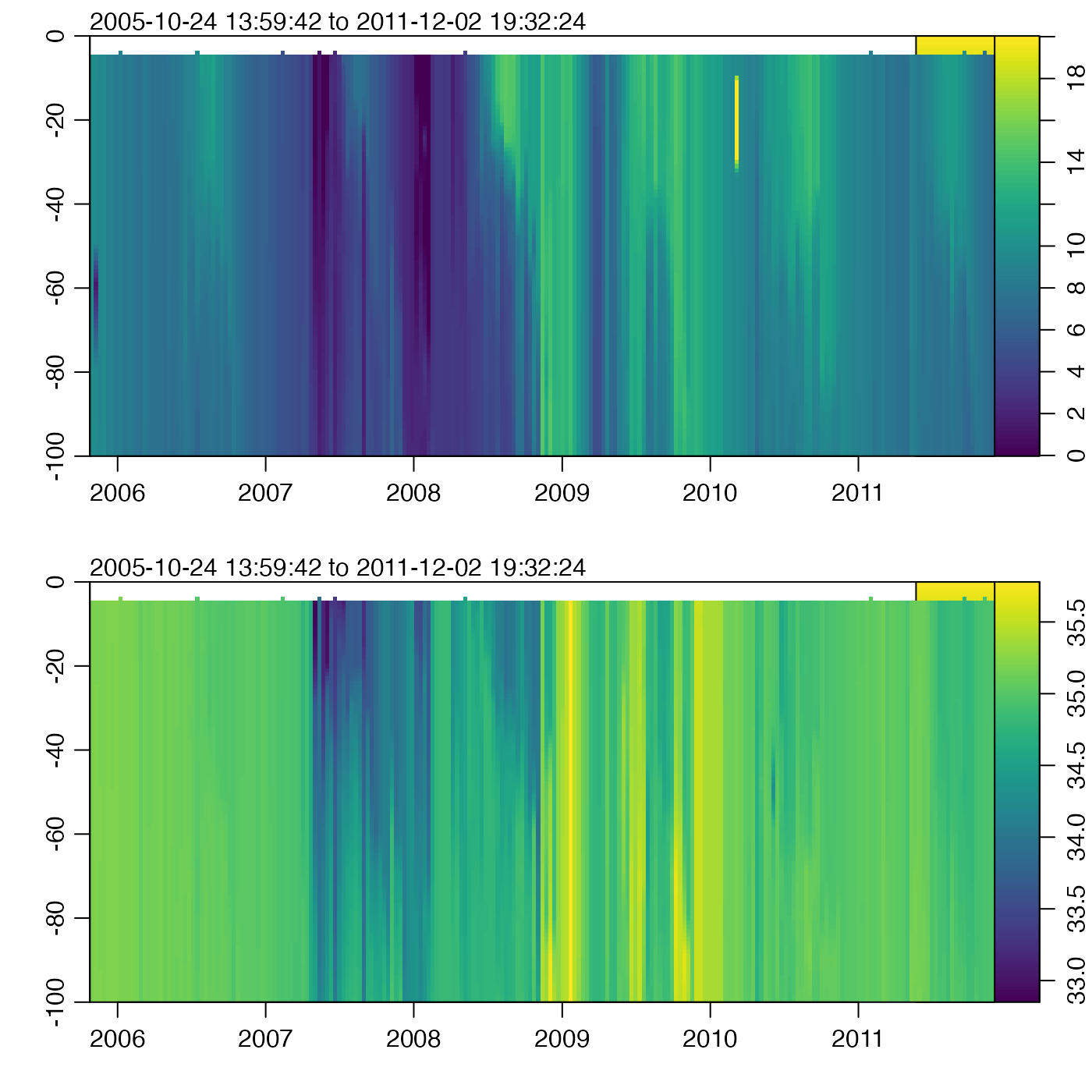Grid an Argo float, by interpolating to fixed pressure levels. The gridding is done with approx(). If there is sufficient user demand, other methods may be added, by analogy to sectionGrid().

argoGrid(argo, p, debug = getOption("oceDebug"), ...)

## Arguments

argo

A argo object to be gridded.

p

Optional indication of the pressure levels to which interpolation should be done. If this is not supplied, the pressure levels will be calculated based on the existing values, using medians. If p="levitus", then pressures will be set to be those of the Levitus atlas, given by standardDepths(), trimmed to the maximum pressure in argo. If p is a single numerical value, it is taken as the number of subdivisions to use in a call to seq() that has range from 0 to the maximum pressure in argo. Finally, if a vector numerical values is provided, then it is used as is.

debug

A flag that turns on debugging. Higher values provide deeper debugging.

...

Optional arguments to approx(), which is used to do the gridding.

## Value

x an argo object.

Data-quality flags contained within the original object are ignored by this function, and the returned value contains no such flags. This is because such flags represent an assessment of the original data, not of quantities derived from those data. This function produces a warning to this effect. The recommended practice is to use handleFlags() or some other means to deal with flags before calling the present function.

Other things related to argo data: [[,argo-method, [[<-,argo-method, argo-class, argoNames2oceNames(), argo, as.argo(), handleFlags,argo-method, plot,argo-method, read.argo.copernicus(), read.argo(), subset,argo-method, summary,argo-method

## Author

Dan Kelley and Clark Richards

## Examples

library(oce)
data(argo)
g <- argoGrid(argo, p=seq(0, 100, 1))
par(mfrow=c(2,1))
t <- g[["time"]]
z <- -g[["pressure"]][,1]
# Set zlim because of spurious temperatures.
imagep(t, z, t(g[['temperature']]), ylim=c(-100,0), zlim=c(0,20))
imagep(t, z, t(g[['salinity']]), ylim=c(-100,0))